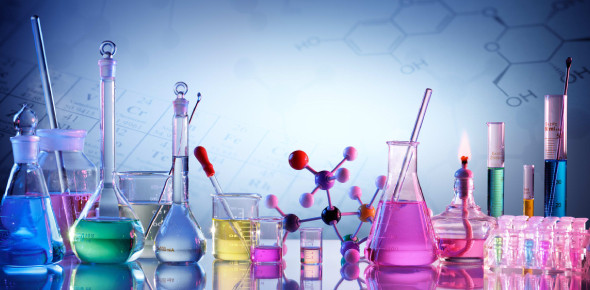# Solutions In Chemistry: MCQ Quiz!

10 Questions | Total Attempts: 1868SettingsThis Chemistry quiz has been designed to test their knowledge and understanding of the concepts involved with the matter, chemical solutions, and reaction. There is a list of 10 questions related to chemical solutions and reactions. Read the questions carefully and answer.

• 1.
A solvent is...
• A.

A solution

• B.

What the solvent is disolved in.

• C.

Is always water.

• D.

Can be a solid.

• E.

B and D

• 2.
What type of mixture is a solution?
• A.

Heterogeneous

• B.

Homogeneous

• C.

Immiscible

• D.

All of the above

• 3.
What is the term that describes the dissolving process?
• A.

Saturation

• B.

Solubulity

• C.

Solvation

• D.

None

• 4.
When two liquids mix, they are said to be...
• A.

Soluble

• B.

Miscible

• C.

Solution

• D.

Solvent

• 5.
Which way is NOT a way to increase the rate of solvation?
• A.

Agitation

• B.

Increased temperature

• C.

increased surface area

• D.

Cooling

• 6.
Solubility is the term that describes the ability of a substance to dissolve at particular temperatures. Solubility is expressed as grams of solute per ______________ grams of solvent.
• A.

10

• B.

50

• C.

100

• D.

200

• 7.
What does it mean when a solution is unsaturated?
• A.

Too much solute

• B.

Not enough solute

• C.

Just enough solute

• D.

None

• 8.
What does it mean when a solution is supersaturated?
• A.

Too much solute

• B.

Not enough solute

• C.

just enough solute

• D.

None

• 9.
Insoluble means that two substances can dissolve in one another.
• A.

True

• B.

False

• 10.
In a solution containing water and polar solute particles _____
• A.

The polar particles will disperse randomly throughout the water

• B.

He polar particles form ionic bonds with water molecules

• C.

The polar particles will not dissolve

• D.

Hydrogen bonding allows the particles to dissolve

Related TopicsBack to top